# Mathway

Mathway Download

## Description

With millions of users and billions of problems solved, Mathway is the world's #1 math problem solver. From basic algebra to complex calculus, Mathway instantly solves your most difficult math problems - simply type your problem in (or point your camera and snap a pic!) to receive instant free answers. Need detailed step-by-step solutions? Mathway is like a private tutor in the palm of your hand, providing instant homework help anywhere, anytime.

Mathway covers:
- Basic Math
- Pre-Algebra
- Algebra
- Trigonometry
- Precalculus
- Calculus
- Statistics
- Finite Math
- Linear Algebra
- Chemistry
- Graphing

Got a math problem? Just ask Mathway.

"Easy to use and efficient, Mathway appeals to anyone who needs help solving mathematical problems, whether we're talking about high-school students or undergraduates." - Yahoo! News

"If you've got some math problems that need solving, check out Mathway. This tool will show you how it reached its answer, letting you learn from the process." - CNET

"Mathway is an irreplaceable tool when it comes to problem solving. The app helps you do your maths homework. Not only does it do your assignment for you, it teaches you how to do it right. All you have to do is put the equation in and press the Enter button." - Lifehack

## Screenshots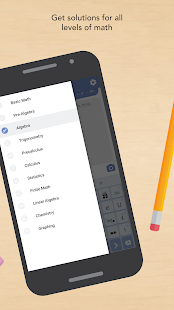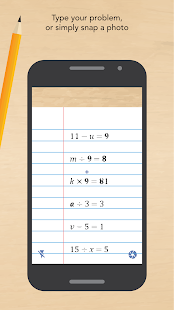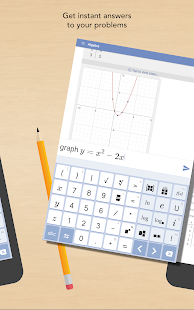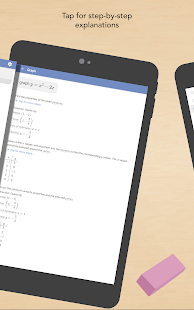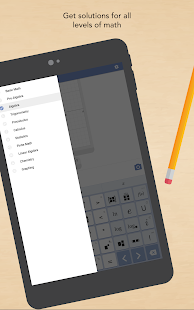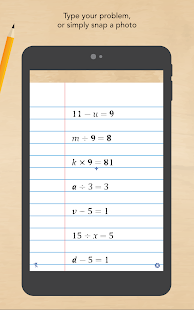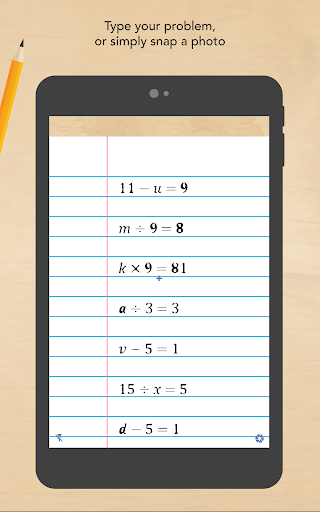## Downloads

Download cracked APK of Mathway ( com.bagatrix.mathway.android )

## Comments

No Comments yet.
You have to login
27512 Apps online